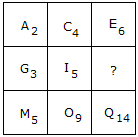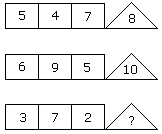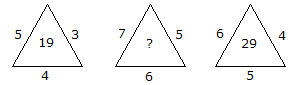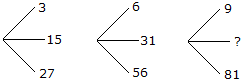# Verbal Reasoning - Character Puzzles

## Why Verbal Reasoning Character Puzzles?

In this section you can learn and practice Verbal Reasoning Questions based on "Character Puzzles" and improve your skills in order to face the interview, competitive examination and various entrance test (CAT, GATE, GRE, MAT, Bank Exam, Railway Exam etc.) with full confidence.

## Where can I get Verbal Reasoning Character Puzzles questions and answers with explanation?

IndiaBIX provides you lots of fully solved Verbal Reasoning (Character Puzzles) questions and answers with Explanation. Solved examples with detailed answer description, explanation are given and it would be easy to understand. All students, freshers can download Verbal Reasoning Character Puzzles quiz questions with answers as PDF files and eBooks.

## Where can I get Verbal Reasoning Character Puzzles Interview Questions and Answers (objective type, multiple choice)?

Here you can find objective type Verbal Reasoning Character Puzzles questions and answers for interview and entrance examination. Multiple choice and true or false type questions are also provided.

## How to solve Verbal Reasoning Character Puzzles problems?

You can easily solve all kind of Verbal Reasoning questions based on Character Puzzles by practicing the objective type exercises given below, also get shortcut methods to solve Verbal Reasoning Character Puzzles problems.

### Exercise :: Character Puzzles - Character Puzzles 1

1.

Which one will replace the question mark ?A. L10 B. K15 C. I15 D. K8

Explanation:How the number is obtained?

2 + 4 = 6

5 + 9 = 14

Similarly,

3 + 5 = 8

Therefore, the answer is K8.

2.

Which one will replace the question mark ?A. 1 B. 4 C. 3 D. 6

Explanation:

(5 + 4 + 7)/2 = 8

(6 + 9 + 5)/2 = 10

(3 + 7 + 2)/2 = 6.

3.

Which one will replace the question mark ?A. 18 B. 12 C. 9 D. 6

Explanation:

(12 + 18 + 30)/10 = 6

(16 + 24 + 40)/10 = 8

Similarly, (45 + 18 + 27)/10 = 9.

4.

Which one will replace the question mark ?A. 25 B. 37 C. 41 D. 47

Explanation:

(5 x 3) + 4 = 19

and (6 x 4) + 5 = 29

Therefore, (7 x 5) + 6 = 41

5.

Which one will replace the question mark ?A. 45 B. 41 C. 32 D. 40

Explanation:

(15 x 2 - 3) = 27,

(31 x 2 - 6) = 56

and (45 x 2 - 9) = 81

#### Current Affairs 2022

Interview Questions and Answers